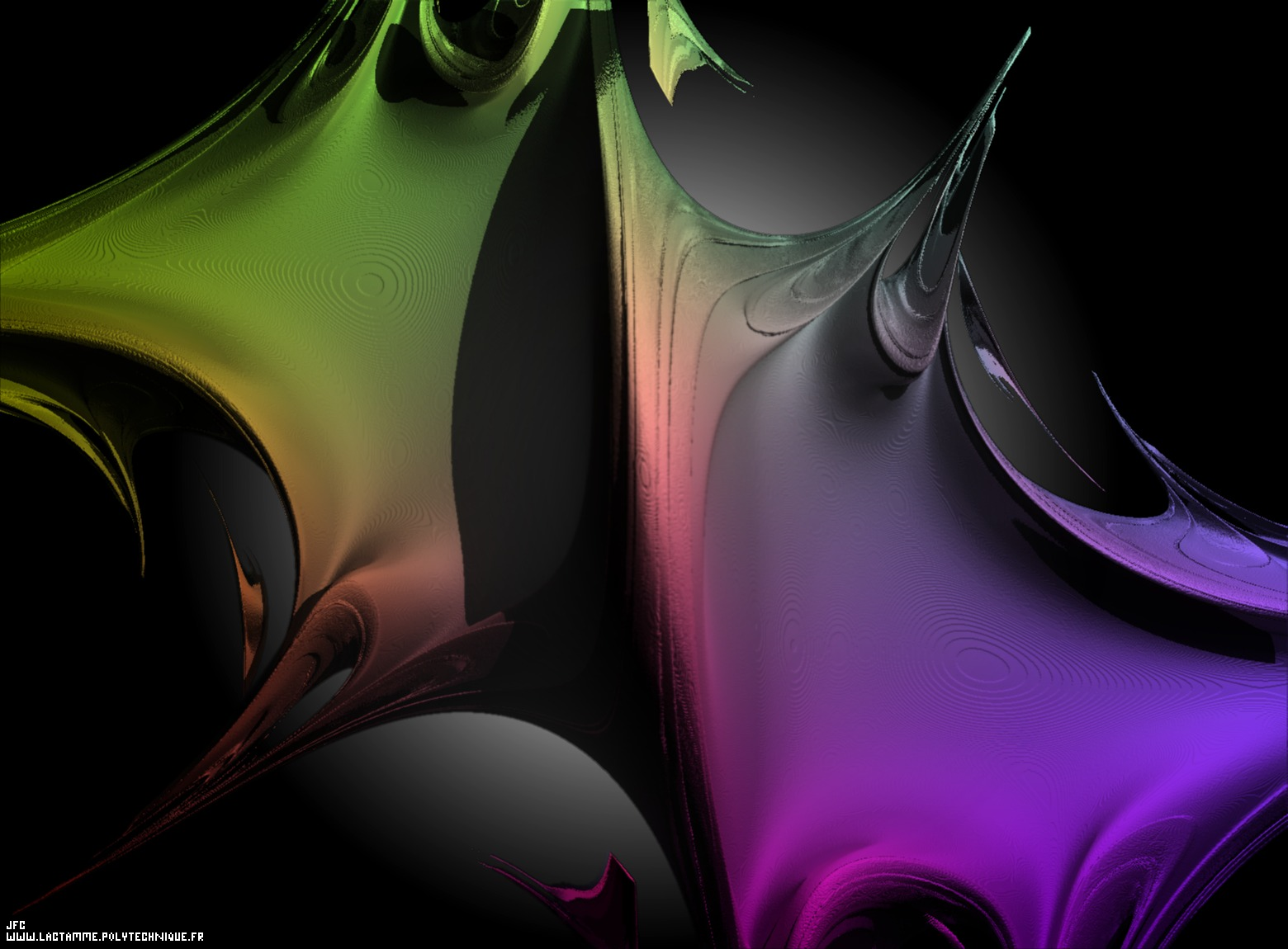The quaternionic Julia set -degree=2- computed -pseudo-addition and pseudo-multiplication in C- with A=(-0.58...,+0.63...,0,0) -tridimensional cross-section- [L'ensemble de Julia -degré=2- dans le corps des quaternions calculé -pseudo-addition et pseudo-multiplication dans C- pour A=(-0.58...,+0.63...,0,0) -section tridimensionnelle-].

The quaternionic numbers are defined by means of the complex numbers. Then the addition and the multiplication of quaternionic numbers are defined by means of the addition and the multiplication of complex numbers. Let's recall that the addition ('+') of two complex numbers z1={x1,y1} and z2={x2,y2} is defined as:
```                    z1+z2 = {x1,y1}+{x2,y2} = {x1+x2,y1+y2}
```
when the multiplication ('*') is a little more complicated:
```                    z1*z2 = {x1,y1}*{x2,y2} = {x1*x2 - y1*y2,x1*y2 + x2*y1}
```
Even if it is a mathematical non sense, one can try more complicated definitions (the so-called pseudo-addition and pseudo-multiplication):
```                    z1+z2 = A(x1,y1,x2,y2)
```
```                    z1*z2 = M(x1,y1,x2,y2)
```
'A' and 'M' being two arbitrary complex functions, for example:
```                    A(x1,y1,x2,y2) = {x1+x2+(alpha*x1*y1),y1+y2+(beta*x2*y1)}
```
('alpha' and 'beta' being two arbitrary real numbers) and:
```                    M(x1,y1,x2,y2) = {x1*x2 - y1*y2,x1*y2 + x2*y1}
```
(the multiplication being unchanged in this example...).

See some related pictures of quaternionic Julia sets (possibly including this one):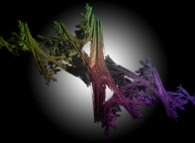alpha=beta=0(standard addition and standard multiplication)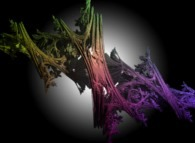alpha=beta=0.1(pseudo-addition and standard multiplication)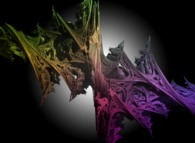alpha=beta=0.2(pseudo-addition and standard multiplication)alpha=beta=0.3(pseudo-addition and standard multiplication)alpha=beta=0.4(pseudo-addition and standard multiplication)alpha=beta=0.5(pseudo-addition and standard multiplication)

See some related pictures of quaternionic Mandelbrot sets (possibly including this one):alpha=beta=0(standard addition and standard multiplication)alpha=beta=0.1(pseudo-addition and standard multiplication)alpha=beta=0.2(pseudo-addition and standard multiplication)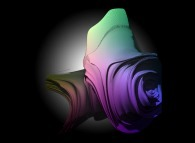alpha=beta=0.3(pseudo-addition and standard multiplication)alpha=beta=0.4(pseudo-addition and standard multiplication)alpha=beta=0.5(pseudo-addition and standard multiplication)

But more "complex" definitions can be used. For example, each of the real and imaginary parts of 'A' and 'M' can be defined with something like:
```                    (((((((a1111*x1)+a1110)*y1)+((a1101*x1)+a1100))*x2)+((((a1011*x1)+a1010)*y1)+((a1001*x1)+a1000)))*y2)+((((((a0111*x1)+a0110)*y1)+((a0101*x1)+a0100))*x2)+((((a0011*x1)+a0010)*y0001)+((a1*x1)+a0000)))
```
('annnn' denoting arbitrary real numbers).

See some other related pictures of quaternionic Julia sets (possibly including this one):See three other pictures of quaternionic Julia sets: the first one -left-hand side- is obtained by means of the standard addition and the standard multiplication. The two next ones are computed with all real numbers 'annnn' being random (inside [-0.01,+0.01] and [-0.02,+0.02] respectively) except those defining the standard addition ({a0010=+1,a0001=+1} for the real part and {a1000=+1,a0100=+1} for the imaginary one) and the standard multiplication ({a1100=-1,a0011=+1} for the real part and {a1001=+1,a0110=+1} for the imaginary one):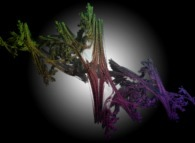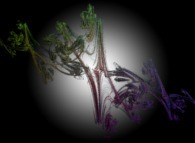(CMAP28 WWW site: this page was created on 12/16/2018 and last updated on 09/02/2022 10:43:47 -CEST-)

[See all related pictures (including this one) [Voir toutes les images associées (incluant celle-ci)]]

[Please visit the related DeterministicFractalGeometry picture gallery [Visitez la galerie d'images DeterministicFractalGeometry associée]]

[Go back to AVirtualMachineForExploringSpaceTimeAndBeyond [Retour à AVirtualMachineForExploringSpaceTimeAndBeyond]]

[The Y2K Bug [Le bug de l'an 2000]]

[Site Map, Help and Search [Plan du Site, Aide et Recherche]]
[Mail [Courrier]]
[About Pictures and Animations [A Propos des Images et des Animations]]